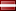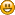Author Topic: Multivariate linear regression  (Read 1741 times)AndrewF

• Jr. Member
•• Posts: 15Multivariate linear regression
« on: May 05, 2014, 12:09:35 PM »
I need to implement an algorithm to calculate multivariate linear regression that when I call in C the assembly written code returns me the result.

E.g.

Code: [Select]
`mylinregNasm(Xy, result, m, n)`;

Where result is
Code: [Select]
`VECTOR result= get_block(sizeof(float),n);`
Xy is a matrix of size m × n +1) in which the first n columns represent the independent variables x1, ..., xn and the last column represents the dependent variable y; each row contains one observation (in the first n +1 columns) and the associated value of the dependent variable (last column). The output is an array of n columns containing the coefficients of the regression hyperplane.

Could some skilled NASM developer help me?encryptor256

• Full Member
•• Posts: 250
• Country:• Win64 .Re: Multivariate linear regression
« Reply #1 on: May 05, 2014, 12:42:47 PM »
Your idea is not clear and foggy, you forgot to tell something more.

Current dialog is this:

Quote
I need to implement an algorithm

Cool, then start doing it!

Quote
multivariate linear regression

What is that? Looks like copied from some science book, which doesn't make sense at all.

Quote
mylinregNasm(Xy, result, m, n)

What is that? Doesn't looks like C or Assembly.

Quote
VECTOR result= get_block(sizeof(float),n);

What is VECTOR and get_block?

Quote
Could some skilled NASM developer help me?

With what? Maybe let's start with rewriting all of that what you actually want to accomplish.

Do really someone would like to help you, with that kind'a task description?Hmm.... Let me think.. NO!